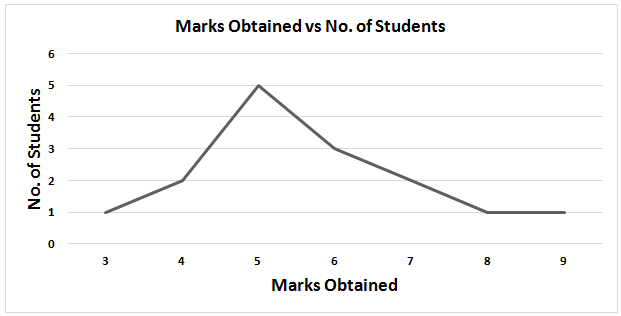# Symmetry and Skewness in returns Distribution

## Introduction

• Data is the most powerful around the globe, it has become powerful and valuable than the oil. Raw data is of no use until it gives some information. To obtain information from data, it should be well sorted to do any kind of analysis.
• There are many ways to sort and represent data like pictorial representation using charts, frequency distribution charts, etc.
• The measure of Central Tendency is operations that are performed on a set of data to obtain the center of a dataset i.e. where most of the values lie. Mean is the average of values in a data set, Median is the value of the middle element of data set that has been sorted in ascending or descending order and Mode is the element in the dataset that has the highest frequency.
• Dispersion is a measure to find out the extent to which values on elements differ from the Mean of the dataset. Standard Deviation the most widely used method to measure the Dispersion of a set of data. It measures the deviation of elements of a population or dataset from its mean. Standard Deviation is calculated as the positive square root of the mean of squared deviations from the mean.

## Symmetric/Normal Distribution

• Symmetric Distribution is one in which frequencies of the elements of the dataset are evenly/equally distributed around the Median or a dataset is said to be symmetric if it looks the same to the left and right of the center point. The following Illustrations will clarify the statement.

#### Illustration 1:

• 3,4,5,8,5,9,6,7,8,7,6,7,5,5,7,6,6,5,6,7,4,6,4,6,8 are the marks obtained by students of a class. Let us create its Frequency Distribution.
Frequency Distribution
Marks No. of Students
3 1
4 3
5 5
6 7
7 5
8 3
9 1
• Median of the Dataset = 6, Mode of the Dataset = 6, Mean of Dataset= 6
• Hence, Mean = Median = Mode
• If we plot a graph of Marks Obtained vs Number of Students, it will look like,• From the above graph, it seems that it is symmetric on the Median of dataset i.e. 6, each side of distribution is a mirror image of the other. In simple words, the number of students who got more than 6 marks is equal to the number of students who got less than 6 marks.

#### Illustration 2:

• 3,5,4,5,4,5,6,7,5,6,7,6,5,8,9 are the marks obtained by a class in a subject. Let us create the frequency distribution.
Frequency Distribution
Marks No. of Students
3 1
4 2
5 5
6 3
7 2
8 1
9 1
• Median of the Dataset = 5, Mode of the Dataset = 5, Mean of Dataset= 5.67• From the above graph, the graph is not symmetric on its Median i.e. 5, the two sides of the median are not a mirror image of each other, hence it is not asymmetric distribution.
• Also, Median = Mode ≠ Mean

## Important Points (Symmetric Distribution)

• The elements are well-distributed around the Mean.
• Mean = Median = Mode will always hold true for a Symmetric Distribution.
• Around 68% of the elements lie around plus and minus one standard deviation, 95% lie around plus minus two standard deviations, and 99% lie around plus-minus 3 standard deviations.

## Skewed Distribution

• A Skewed Distribution is just the opposite of Symmetric Distribution. In this distribution, the frequencies are not well spread around the median. Let us see the graph for illustration 2 again.• The Median in the dataset is around 5, but the frequencies are not well distributed on either side of the median. The number of students who got greater than 5 marks is more in number as compared to students who got less than 5 marks.
• For a Skewed Distribution Mean and Median can never be equal.

## Types of Skewed Distribution

• Positively Skewed Distribution: In a distribution, if most of the values are clustered in the left-half, then it is known as Positively Skewed or Right Skewed Distribution. E.g. The graph obtained in Illustration 2 above is a Positively Skewed Distribution.
• Negatively Skewed Distribution: In a distribution, if most of the values are clustered in the right-half, then it is known as Negatively Skewed or Left Skewed Distribution.